## Example Questions

← Previous 1 3

### Example Question #121 : Integers

The product of two consecutive positive integers is 272. What is the larger of the two integers?

17

19

18

16

15

17

Explanation:

In order to multiply to 272, the units digits of the two integers will have to multiply to a number with a units digit of 2. For 17, you can see that 6 x 7 (the units digits of 16 and 17) = 42. The best strategy here is to plug in the choices using the units digit strategy.

### Example Question #122 : Integers

What is the units digit of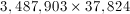?Explanation:

The units digit of any product depends on the units digits of the 2 numbers multiplied, which in this case is 3 and 4. Since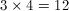, the units digit ofis 2.

### Example Question #123 : Integers

What is the units digit of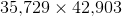Explanation:

The units digit of the product of any two numbers is the same as the units digit of the product of the two numbers' units digits. In this case, it would be the units digit of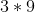, which is.

### Example Question #124 : Integers

Choose the answer below which best solves the following equation: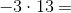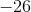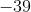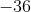Explanation:

When multiplying integers, if one of the integers is negative, your answer will be negative:

First multiply the ones digits together.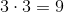Next multiply the ones digit of the smaller number with the tens digit of the larger number to get the tens digit of the product.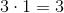Combining these two and remembering the negative sign we get our final answer: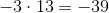### Example Question #125 : Integers

Choose the answer which best solves the following equation: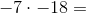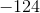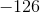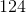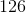Explanation:

When multiplying integers, if both of the signs of the integers are the same (positive, or negative) then your result will be positive:

First multiply the ones digit of both numbers together. If the product is greater than ten remember to carry the one to the tens place.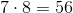Next multiply the ones digit of the smaller number with the tens digit of the larger number and add the number that was carried over.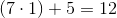Combine these two together to get: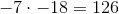### Example Question #126 : Integers

Of a group of 335 graduating high school atheletes, 106 played basketball, 137 ran track and field, and 51 participated in swimming. What is the maximum number of students that did both track and field and swimming upon graduation?

335

106

51

55

157

51

Explanation:

Simply recognize that logically, the participation of either sport is non-exclusive, that is, just because people took track and field does not necessarily mean they did not take swimming as well. As such, those 51 who took swimming could have all potentially done track and field, which means all 51 students.

### Example Question #127 : Integers

Of 300 students, 120 are enrolled in math club, 150 are enrolled in chess club, and 100 are enrolled in both. How many students are not members of either club?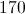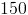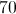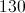Explanation:

There are 120 students in the math club and 150 students in the chess club, for a total membership of 270. However, 100 students are in both clubs, which means they are counted twice. You simply subtract 100 from 270, which will give you a total of 170 different students participating in both clubs. This means that the remaining 130 students do not participate in either club.

### Example Question #128 : Integers

Choose the answer which best solves the following equation: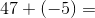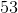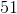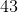Explanation:

When adding integers, one needs to pay close attention to the sign.  When you add a negative integer, it's the same thing as subtracting that integer.  Therefore: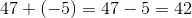### Example Question #129 : Integers

Choose the answer below which best solves the following equation: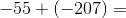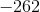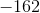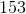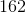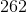Explanation:

The sum of any two negative numbers will be negative.  Remember, also, that adding a negative number is like subtracting it.  Therefore: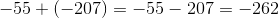### Example Question #1 : Operations

Apples are sold by whole bushels. You cannot purchase part of a bushel. There are 126 apples in a bushel.

Sam is a caterer who needs to bake 300 pies to sell at the county fair. If it takes 4 apples to make a pie, how many bushels must Sam order to ensure he has enough apples for his pies?

11

10

9

12

9.5

10

Explanation:

Because Sam needs to make 300 pies, and each pie needs 4 apples, the number of apples he needs is

300 x 4 = 1200.

To determine how many bushels Sam needs, divide the total number of apples by the number of apples sold in a bushel.

1200 / 126 = 9.524

Because apples are sold by the whole bushel, Sam cannot order part of a bushel. In order to make sure he has sufficient apples, he will need to order 10 bushels.

← Previous 1 3

Tired of practice problems?

Try live online GRE prep today.